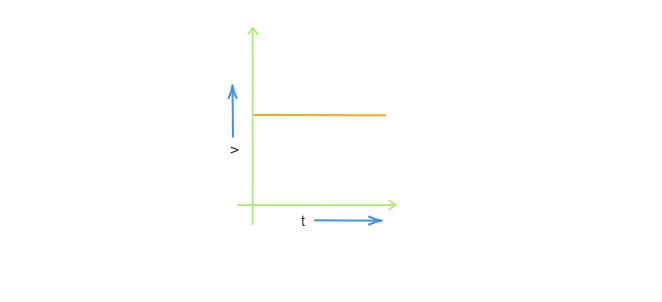# From the given $v-t$ graph it can be inferred that the object is$(a)$. in uniform motion$(b)$. at rest$(c)$. in non-uniform motion$(d)$. moving with uniform acceleration"

In the given $v-t$ graph, it is a straight line parallel to $t-axis$ which indicates that the velocity is the same with respect to the time interval. So the object is moving in uniform motion.

So, option $(a)$ is correct.